Let’s continue our work on image alignment. We shall cover further details on image warping.

For what comes next, we’ll work a bit in Python. Import the following packages :

import cv2
import numpy as np
from matplotlib import pyplot as plt


So far, we saw how to :

• detect features (Harris corner detector, Laplacian of Gaussians for blobs, difference of Gaussians for fast approximation of the LOG)
• the properties of the ideal feature
• the properties of the feature descriptor
• matching of local features
• feature distance metrics

We’ll cover into further details image alignment.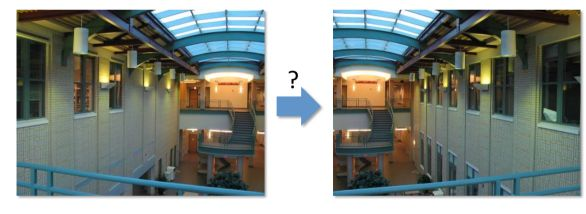# I. Image Warping

There is a geometric relationship between these 2 images :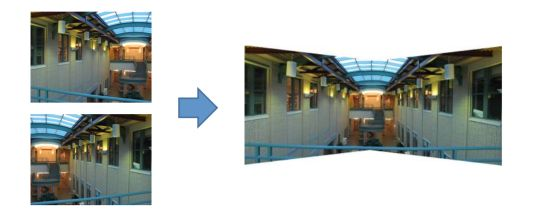An image warping is a change of domain of an image : $$g(x) = f(h(x))$$. This might include translation, rotation or aspect change. These changes are said to be global parametric warping: $$p' = T(p)$$, since the transformation can easily be described by few parameters and is the same for every input point.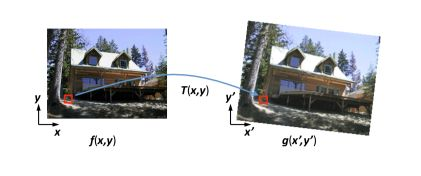To build the transformed image, we usually apply an inverse-warping :

• for every pixel $$x'$$ in $$g(x')$$ :
• compute the source location $$x = \hat{h}(x')$$
• resample $$f(x)$$ at location $$x$$ and copy to $$g(x')$$

This allows $$\hat{h}(x')$$ to be defined for all pixels in $$g(x')$$.

## 1. Linear transformations

We’ll now cover the different types of linear transformations that we can apply to an image using inverse-warping.

### a. Uniform Scaling

Scaling by factor $$s$$ :

$S = \begin{pmatrix} s & 0 \\ 0 & s \end{pmatrix}$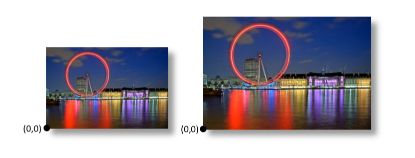### b. Rotation

Rotation by angle $$\theta$$ :

$R = \begin{pmatrix} cos \theta & - sin \theta \\ sin \theta & cos \theta \end{pmatrix}$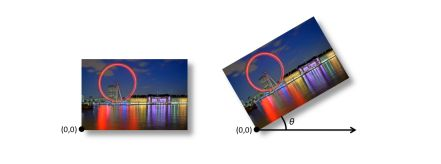### c. 2D Mirror about the Y-axis

$T = \begin{pmatrix} -1 & 0 \\ 0 & 1 \end{pmatrix}$

### d. 2D Miror accross line $$y = x$$

$T = \begin{pmatrix} 0 & 1 \\ 1 & 0 \end{pmatrix}$

### e. All 2D linear transformations

In summary, the linear transforms we can apply are :

• scale
• rotation
• shear
• mirror
$\begin{pmatrix} x' \\ y \end{pmatrix} = \begin{pmatrix} a & b \\ c & d \end{pmatrix} \begin{pmatrix} x \\ y \end{pmatrix}$

The transformation should respect the following properties :

• origin maps origin
• lines map to lines
• parallel lines remain parallel
• ratios are preserved
• closed under composition

## 2. Translation

The trick is to add one more coordinate to build homogenous image coordinates.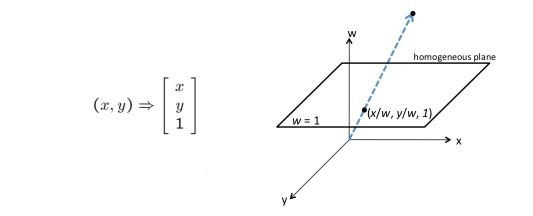## 3. Affine transformation

An affine transformation is any transformation that combines linear transformations and translations. For example :

$\begin{pmatrix} x' \\ y' \\ w' \end{pmatrix} = \begin{pmatrix} a & b & c \\ d & e & f \\ 0 & 0 & 1 \end{pmatrix} \begin{pmatrix} x \\ y \\ w \end{pmatrix}$

In affine transformations, the origin does not always have to map the origin.

## 4. Homography

The Homography transform is also called projective transformation or planar perspective map.

$H = \begin{pmatrix} a & b & c \\ d & e & f \\ g & h & 1 \end{pmatrix}$

Homographic transformations simply respect the following properties :

• lines map to lines
• closed under composition

The different transformations can be summarized this way :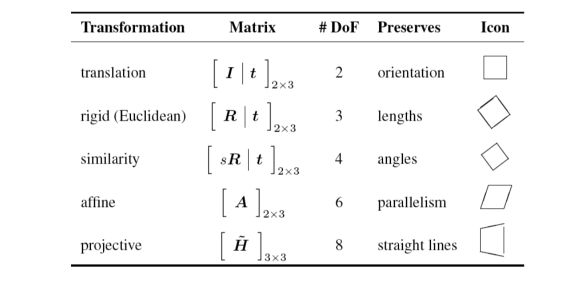With homographies, points at infinity become finite vanishing points.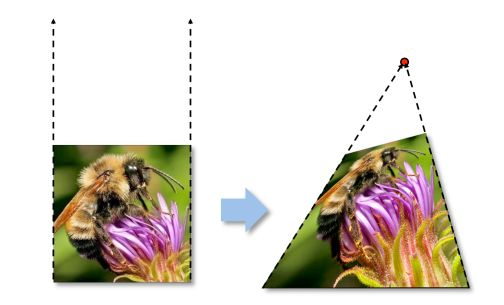# II. Computing transformations

Given a set of matches between images $$A$$ and $$B$$, we must find the transform $$T$$ that best agrees with the matches.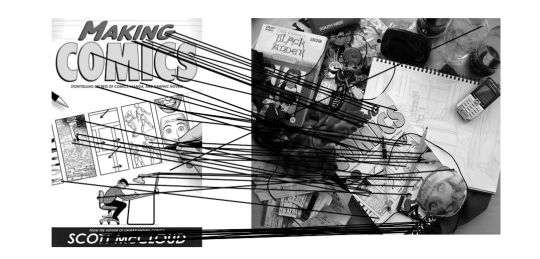## 1. Translation

The displacement of match $$i$$ is $$(x_i' - x_i, y_i' - y_i)$$ where $$x_i' = x_i + x_t$$ and $$y_i' = y_i + y_t$$. We want therefore to solve :

$(x_t, y_t) = ( \frac {1} {n} \sum_i x_i' - x_i, \frac {1} {n} \sum_i y_i' - y_i )$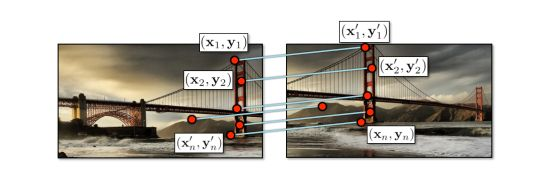We face an overdetermined system of equations, which can be solved by least squares.

$r_{x_i}(x_t) = x_i + x_t - x_i'$ $r_{y_i}(y_t) = y_i + y_t - y_i'$

The goal is to minimize the sum of squared residuals :

$C(x_t, y_t) = \sum_i (r_{x_i}(x_t)^2 + r_{y_i}(y_t)^2)$

We can rewrite the problem in matrix form :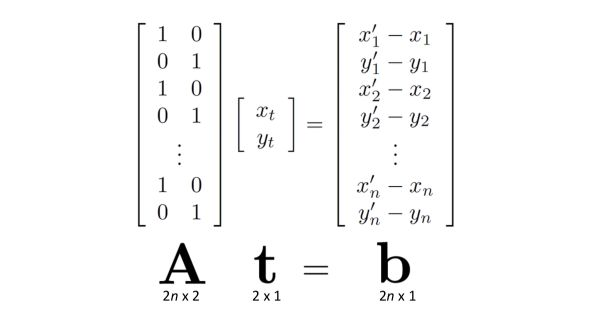And the solution heads : $$t = (A^T A)^{-1} Ab$$

## 2. Affine transformation

$\begin{pmatrix} x' \\ y' \\ w' \end{pmatrix} = \begin{pmatrix} a & b & c \\ d & e & f \\ 0 & 0 & 1 \end{pmatrix} \begin{pmatrix} x \\ y \\ w \end{pmatrix}$

We can write the residuals as :

$r_{x_i}(a,b,c,d,e,f) = (ax_i + by_i + c) - x_i'$ $r_{y_i}(a,b,c,d,e,f) = (dy_i + ey_i + f) - y_i'$

And rewrite the cost function as :

$C(a,b,c,d,e,f) = \sum_i ( r_{x_i}(a,b,c,d,e,f)^2 + r_{y_i}(a,b,c,d,e,f)^2 )$

Which can be rewritten in matrix form as :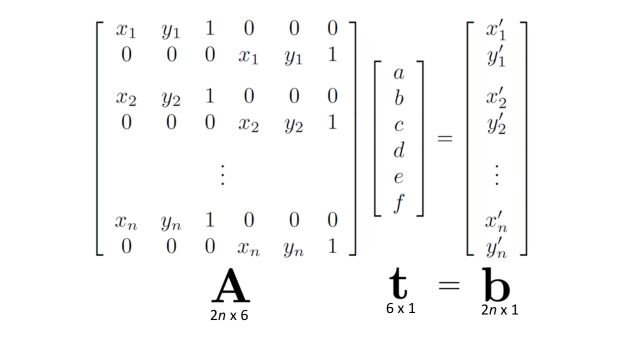Let’s develop a more general formulation. We have $$x' = f(x,p)$$ a parametric transformation. The Jacobian of the transformation $$f$$ with respect to the motion parameters $$p$$ determines the relationship between the amount of motion $$\Delta x = x' - x$$ and the unknown parameters $$\Delta x = x' - x = J(x)p$$ . We note that $$J = \frac {\delta f} {\delta p}$$.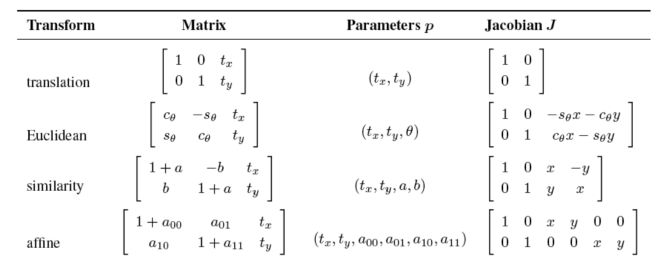The sum of squared residuals is then :

$E_{LLS} = \sum_i {\mid \mid J(x_i)p - \Delta x_i \mid \mid_2 }^2$

The solution yields :

$$Ap = b$$ where :

• we define $$A = \sum_i J^T(x_i)J(x_i)$$ the Hessian
• and $$b = \sum_i J^T(x_i) \Delta x_i$$

Up to now, we considered only a perfect matching accuracy. It’s however only rarely the case. We can weight the least squares problem :

$$E_{WLS} = \sum_i {\Sigma_i}^2 \mid \mid r_i \mid \mid$$ . If the $$\delta_i$$ are fixed, the solution to apply is : $$p = ( \sum^T A^T A \Sigma)^-1 \sigma^T Ab$$ with $$\Sigma$$ a matix containing for each observation the noise level.

**Conclusion **: I hope this article on image alignment, transformations, and warping was helpful. Don’t hesitate to drop a comment if you have any question.